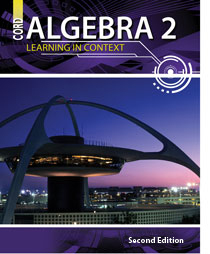# Algebra 2 - 2nd Edition

## Chapter 1: Fundamentals of Algebra

Some links are repeated for use with more than one lesson.

### 1.1 Real Numbers and Properties

http://www.mcs.surrey.ac.uk/Personal/R.Knott/Fractions/FractionsCalc.html
This page contains a fraction calculator that defines whether a fraction terminates or repeats and will show as many decimal places as you request.

http://www.intmath.com/numbers/numbers-intro.php

http://www.regentsprep.org/Regents/math/ALGEBRA/AN1/indexAN1.htm
This page includes links to several summaries of basic number properties and offers hints for remembering them. The page also includes pages with practice problems.

http://www.college-cram.com/study/algebra/presentations/96

### 1.2 Solving Equations and Inequalities

http://www.math.com/school/subject2/lessons/S2U3L1GL.html

http://www.aaaknow.com/equ.htm
This series of pages contains lessons on solving all types of equations and inequalities. (Click Next)

http://www.freemathhelp.com/Lessons/PreAlgebra_Solving_Equations_and_Inequalities_BB.htm
This page has video of a lecture on solving multistep equations and inequalities.

http://www.explorelearning.com/index.cfm?method=cResource.dspResourcesForCourse&CourseID=216
This page contains several different gizmos to practice solving equations and inequalities. This website requires Shockwave Player to run some of its features.

### 1.3 Absolute Value Equations and Inequalities

http://www.themathpage.com/alg/absolute-value.htm
This page contains a lesson on absolute value equations and inequalities. Pass mouse over each colored area to reveal.

http://algebralab.net/studyaids/studyaid.aspx?file=Algebra2_1-7.xml
This page contains a brief explanation and practice on solving absolute value equations and inequalities.

http://www.mathworksheets4kids.com/absolute-value.html
This page contains absolute value practice worksheets of varying levels and operations, including a link to absolute value inequalities worksheets.

http://www.quia.com/cb/37004.html
This page contains a jeopardy-like one or two-player game on solving absolute value equations
and inequalities.

### 1.4 Rate of Change and Linear Equations

http://www.regentsprep.org/regents/math/algebra/ac1/rate.htm

http://math.rice.edu/~lanius/Algebra/stress.html
This page contains a lesson on rate of change with a real life example.

http://www.algebrahelp.com/worksheets/check/graphing/slope.quiz
This page contains an online quiz to calculate slope for several graphs.

http://www.algebra-class.com/rate-of-change.html
This page includes a lesson with several examples and a video about finding the rate of change.

### 1.5 Graphing Linear Equations and Inequalities

http://www.mathsisfun.com/algebra/graphing-linear-inequalities.html

http://www.math.com/school/subject2/lessons/S2U4L3GL.html
This series of pages contains explanation and practice with graphing linear equations. (Click Here)